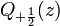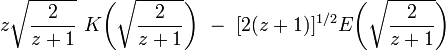User:Tohline/Math/EQ QplusHalf01$~Q_{+\frac{1}{2}}(z)$$~=$$~ z \sqrt{ \frac{2}{z+1} }~K\biggl( \sqrt{ \frac{2}{z+1} } \biggr) ~-~ [2(z+1)]^{1 / 2} E\biggl( \sqrt{ \frac{2}{z+1} } \biggr)$ Abramowitz & Stegun (1995), p. 337, eq. (8.13.7)